| Content Writer: Poornachandra Sarang | Technical Review: Aaditya Damle and ABCOM Team | Copy Editor: Anushka Devasthale | Last Updated: July 23, 2020 Level: Intermediate | Banner image source Internet |

Our world is an interdisciplinary world that consists of numerous fields, and News has to encompass them all. Like old times, the News is not limited to the newspaper anymore, but there is a plethora of information available on social media. Social media circulates News all over the world at the tip of a finger. Staying on top of it all, and staying updated with the ever-changing world is next to impossible.

With so much going on in every corner of the world, how should we manage to keep ourselves updated?Image Source: Internet

Well, here is an option for anyone wanting to stay on top of all the happenings and keep themselves updated. We will build a News Aggregator!

The News Aggregator that we are going to develop in this tutorial will help us in isolating all the News and trends based on our chosen keyword. The keyword can be a geographic location, an organization, a time, or anything. Fortunately, we have an available dataset, which we will be using in training our neural network to build our News Aggregator. In this dataset, there are several predefined sentences with the keywords for these categories already marked out.

Such applications are also useful to the editors of news magazines, where the editor may like to categorize all the articles based on specific sections such as politics, sports, crime, or maybe some other field. You would find many areas where this kind of application will find its use.

Such an application in technical terms is called Name Entity Recognition (NER).

#### Prerequisites:

Knowledge of LSTM (Long Short-Term Memory)

## Application Description

In this project, we will build and train a bidirectional LSTM neural network to recognize named entities in text data. Named entity recognition (NER) models are used to identify mentions of people, locations, organizations, etc. NER is not only a standalone tool for information extraction, but it is also an invaluable preprocessing step for many downstream natural language processing applications like machine translation, question answering, and text summarization.

Generally, the readers can capture important information in the texts such as which word in a sentence is a geographical entity or a political entity based on the tag information. Knowing the relevant tag for each word in a sentence helps in automatically categorizing the sentences in predefined hierarchies. Such applications are useful in classifying content for news providers: A large number of online content accounts in being generated by the news and the publishing houses daily and managing them correctly can be a tedious task for humans. NER can automatically scan the entire articles and help editors in identifying and retrieving major people, organizations, and places discussed in those. As an example, consider the following paragraph:

The U.S geographic government's official line may be that unidentified flying objects (UFOs) don't pose a national security threat, but a group of former Air Force organization officers gathered on Monday in the nation's capital to tell a different story.

In the above paragraph, the entities of three types: Organization, Place, Time, are classified.

By the end of this project, you will be able to build and train a bidirectional LSTM neural network model to recognize the named entities in any textual data.

## Creating a Project

Create a Colab notebook and rename it as NER. Import the required libraries. Do not know how to use Colab? Here is a short tutorial.

``````%matplotlib inline
import matplotlib.pyplot as plt
import pandas as pd
import numpy as np
import tensorflow as tf
from tensorflow.keras.utils import to_categorical
from sklearn.model_selection import train_test_split
from tensorflow.keras import Model, Input
from tensorflow.keras.layers import LSTM, Embedding, Dense
from tensorflow.keras.layers import TimeDistributed, SpatialDropout1D, Bidirectional
from tensorflow.keras.callbacks import ModelCheckpoint, EarlyStopping
``````

You will use the GMB text corpus developed at the University of Groningen. The corpus consists of public domain English texts with corresponding syntactic and semantic representations. The text is tagged to help us identify the named entities such as name, location, etc. Let us first load the data, and then I will explain to you its structure and the various embedded tags.

``````data=pd.read_csv('https://raw.githubusercontent.com/abcom-mltutorials/NEWSAggregator/master/ner_dataset.csv',encoding='latin1')
data=data.fillna(method="ffill")
``````

### Examining Data

Examine the data contents by printing the few records:

``````data.head()
``````

This prints the following output on your screen: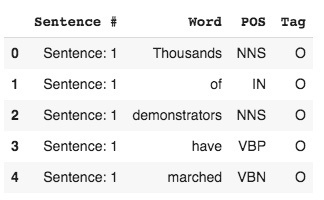Each sentence in the corpus is split into the words. For each word, a tag and POS value are assigned. In the above example, the sentence is

“Thousands of demonstrators have marched …”

Note the first column in the above table says Sentence #. So all the words with Sentence: 1 value will form the first sentence. For the second sentence, the column value would be Sentence: 2 and similarly the rest. Later on, I will show you how to extract the full sentence from this dataset. Before that, let me explain to you the POS and tag columns.

## What is a tag?

Each word is tagged and labeled using the BIO scheme, where each entity label is prefixed with either B or I letter.

B- denotes the beginning and I- inside of an entity.
The words which are not of interest are labeled with 0 – tag. The following table shows a few tags.

Tag Meaning Sample
geo Geographical entity LONDON
org organization New york times
per person Harry
gpe Geopolitical entity European
tim Time indicator Week, Monday
art artifact Economics
eve event olympics
nat Natural phenomenon earthquake
O Other Of , an , the etc

Let us print all the unique tags:

``````data.Tag.unique()
``````

This is the output: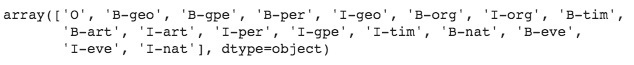### What is POS?

The POS column represents Part Of Speech. The following table shows a few POS tags and their meanings.

Tag Meaning
CC coordinating conjunction
CD cardinal digit
DT determiner
MD modal could, will
NN noun, singular 'desk'
NNS noun plural 'desks'
NNP proper noun, singular 'Harrison'
NNPS proper noun, plural 'Americans'
VB verb, base form take
VBD verb, past tense took
VBG verb, gerund/present participle taking
VBN verb, past participle taken
VBP verb, sing. present, non-3d take

In our project, we will use only the Tag column value and not the POS value as we are interested in classifying only the tagged entities.

## Preprocessing Data

### Extracting a Sentence

I will now show you how to extract the full sentences from the corpus. For this, we will rename our `Sentence #` column to `sentence` so that we can filter out the words.

``````data.columns=['sentence',"Word",'POS',"Tag"]
``````

Let us now extract sentence # 2 and print it out in the console:

``````print("Sentence #2:"," ".join(data[data.sentence=='Sentence: 2'].Word.tolist()))
``````

The output is as shown below: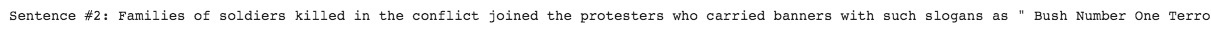Try printing one more sentence. The output is shown below: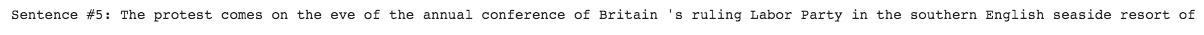We will now make a list of unique words and tags.

### Creating List of Unique Words and Tags

We need a list of unique words and tags for training our neural network. We extract the unique words and tags by creating a Python list.

``````words=list(set(data["Word"].values))
num_words=len(words)
tags=list(set(data["Tag"].values))
num_tags=len(tags)
print ("Number of unique words: ", num_words)
print ("Number of unique tags: ", num_tags)
``````

The number of unique words is 35179 and the number of tags is 17. Note that we have added one tag (ENDPAD) of our own to mark the end of sequences that we will be creating shortly.

## Preparing Data

We need to prepare data in a specific format for machine learning.

### Creating Tuples

For each word, we will create a tuple consisting of Word, POS and Tag (W,P,T). We will create an outer list consisting of these tuples within each sentence. Thus, we define a function called SentenceGetter as follows:def sentencegetter(data):

`````` agg_func=lambda s:[(w,p,t) for w,p,t in zip(s["Word"].values.tolist(),s["POS"].values.tolist(),s['Tag'].values.tolist())]
grouped=data.groupby("sentence").apply(agg_func)
sentences=[s for s in grouped]
return sentences
``````

Now use this function to get a sentence from the corpus.

``````sentences=sentencegetter(data)
``````

You can now see each individual sentence.

``````sentences
``````

The output below shows the first sentence: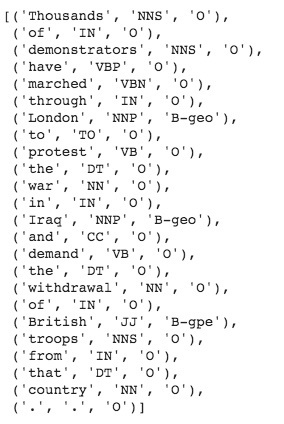### Indexing Words and Tags

As the network understands only the numbers and the text, we need to map each word and a tag to a unique index value. We use a Python dictionary for indexing.

``````word2idx={w:i+1 for i , w in enumerate(words)} #i+1 as we created endpad
tag2idx={t:i for i,t in enumerate(tags)}
tag2idx
``````

Here is the output showing the tag indexed dictionary.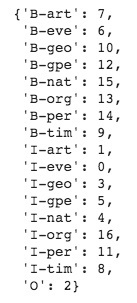We will create fixed-length tensors for model training. To decide on the sequence length, let us first find out the distribution of sentence length in the entire corpus.

``````plt.hist([len(s) for s in sentences],bins=50)
plt.show()
``````

The output is shown below: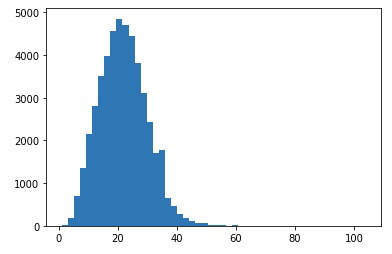As you can see, the maximum length of a sentence is 50. Thus, we will pad all input sequences to a length of 50. It is done in this code:

``````max_len= 50
X= [[word2idx[w] for w in s ] for s in sentences]
``````

Note that before padding, we replace the text words with the corresponding index values. You can see its effect by printing one of the tensors:

``````X
``````

You will see the following output: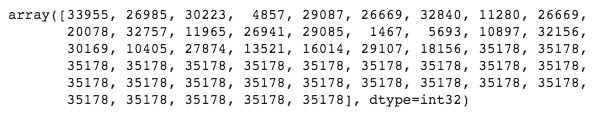Note that each word is replaced with the corresponding index value and the sequence consists of 50 values. After this preprocessing X is our features vector. We need to do similar preprocessing for the target vector. This is done using the following code:

``````#target vector
y=[[tag2idx[w] for w in s ] for s in sentences]
y=[to_categorical(i,num_classes=num_tags) for i in y]
#one hot encoding the target tag
``````

In the above code, I have also done the one hot encoding on the target vector, as we will be using the categorical crossentroy for the loss function.

### Creating Training/Testing Datasets

We split the dataset in a 90:10 ratio for training and testing.

``````xtrain,xtest,ytrain,ytest=train_test_split(X,y,test_size=0.1,random_state=1)
``````

We now build the model.

## Model Building

We build the model using the following code:

``````input_word=Input(shape=(max_len,)) #input layer
model=Embedding(input_dim=num_words,output_dim=max_len,input_length=max_len)(input_word)
model= SpatialDropout1D(0.1)(model) #regularization
# bidirectional for learning across the entire sequence
model=Bidirectional(LSTM(units=100,return_sequences=True,recurrent_dropout=0.1))(model)
out=TimeDistributed(Dense(num_tags,activation="softmax"))(model)
model=Model(input_word,out)
``````

Let us print the model summary. I will then explain to you the purpose of each layer from the summary output.

``````model.summary()
``````

The output is as shown below: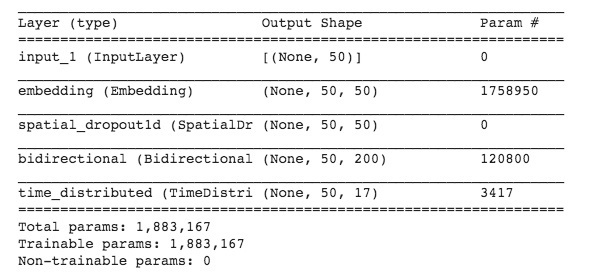I will now explain the purpose of each layer.

Input_word - Input to our model that is nothing but the input sequence of length 50 (max_len).

Embedding layer - This converts positive integers (word counts) into fixed-size dense vectors. It learns the so-called embeddings for a particular text dataset. Embedding layers slowly learn the relationships between words. Hence, if you have a large enough corpus ( which probably contains all possible English words), then vectors for words like "king" and "queen" will show some similarity in the multidimensional space of the embedding. During initialization the following parameters are used:

• 2D input that is input_dim = num of words in the corpus.
• out_dim = maximum length of the sentence
• output 3D tensor with shape: (batch_size, input_length, output_dim).

SpatialDropou1D - This performs the same function as Dropout, however, it drops entire 1D feature maps instead of individual elements. If adjacent frames within feature maps are strongly correlated (as is normally the case in early convolution layers) then regular dropout will not regularize the activations and will otherwise just result in an effective learning rate decrease. In this case, SpatialDropout1D will help promote independence between feature maps and should be used instead.

Bidirectional - Bidirectional LSTMs are an extension of traditional LSTMs that can improve model performance on sequence classification problems. In problems where all time steps of the input sequence are available, Bidirectional LSTMs train two instead of one LSTMs on the input sequence. like it will learn the sequence from both ways from start to end then end to start.

Time Distributed – Time distributed dense layer is used in RNN, including LSTM, to keep one-to-one relations on input and output. Assume you have 60-time steps with 100 samples of data (60 x 100 in other words) and you want to use RNN with an output of 200. If you don't use time distributed dense layers, you will get a 100 x 60 x 200 tensor. So you have the output flattened with each timestep mixed. If you apply the time distributed dense, you are going to apply a fully connected dense on each time step and get output separately by timesteps.

### Model Compiling

We will compile our model using `ADAM` optimizer and `categorical_crossentropy` loss.

``````model.compile(optimizer="adam",loss="categorical_crossentropy",metrics=['accuracy'])
``````

### Model Training

In the model summary, you might have noticed that there are more than 1.8 million trainable parameters. Usually, training LSTMs takes a lot of time. Thus, I will use the early stopping callback so that you may specify a sufficiently large number for the training epoch and the training would automatically stop when your desired accuracy is reached.

``````callbacks=[early_stopping]
history=model.fit(xtrain,np.array(ytrain),validation_split=0.2,batch_size=32,epochs=5,verbose=1,callbacks=callbacks)
``````

This is the output of my run.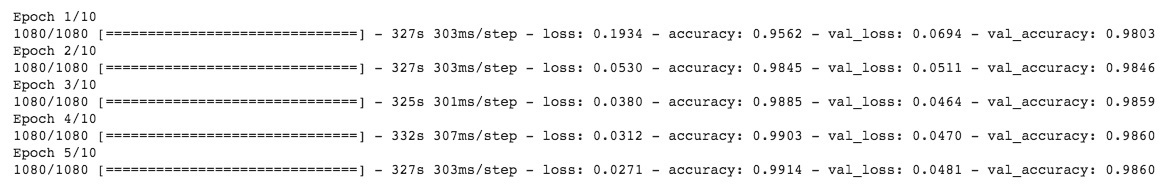Note that the model has stopped training after 5 epochs.

### Model Evaluation

You will evaluate the model’s performance on the test data by calling its evaluation function.

``````model.evaluate(xtest,np.array(ytest))
``````

This was the output in my run: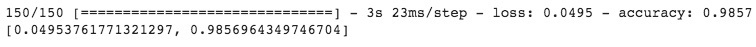We observe 98% accuracy on the test set too.

## Predictions

We will now do a prediction on randomly selected sentence from the test dataset. We will print both the true and predicted values.
We pickup the random sentence:

``````i = np.random.randint(0,xtest.shape)
``````

We now apply the `model.predict` function on the random sentence.

``````p=model.predict(np.array([xtest[i]]))
``````

Since all the target values are one hot encoded and we get the probabilities for each tag. We want to have a tag with the highest probability. To do so we will use `np.argmax` function.

``````p=np.argmax(p,axis=-1)
``````

To get the true value of the prediction for comparison:

``````y_true=np.argmax(np.array(ytest),axis=-1)[i]
``````

Print the prediction results and compare with true values.

``````print("{:15}{:5}\t {}\n".format("word","True","pred"))
print("-"*30)
for w,true,pred in zip(xtest[i],y_true,p):
print("{:15}{}\t{}".format(words[w-1],tags[true],tags[pred]))
``````

This prints the following output on your screen: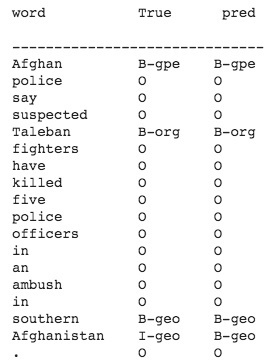From the above result, we can see that the model has correctly predicted tags like Nepalese, Tibetans are geopolitical entities, and of course, Sunday is a time entity.

Now, let us take a sentence that does not exist in a test dataset. Note that for this kind of an unknown statement, we will require the same type of preprocessing, as we did on the test dataset.

Consider the following unseen statement for prediction:

``````sentence="About 1,500 doctors , scientists and health workers are expected to attend the week-long Pan-Africa malaria conference in Cameroon 's capital , Yaounde "
``````

First we convert it into index valued sequence

``````seq=[]
for word in sentence.split():
if word in list(word2idx.keys()):
idx=word2idx[word]
seq.append(idx)
``````

Then we pad it to the maximum length.

``````pad_seq=pad_sequences([seq],padding='post',maxlen=50)
``````

Now apply the predict and argmax function.

``````p=model.predict(pad_seq)
p=np.argmax(p,axis=-1)
``````

Print the predictions:

``````print("{:10}\t {}\n".format("word","pred"))
print("-"*30)
print("{:10}\t{}".format(words[w-1],tags[pred]))
``````

Here is the partial output: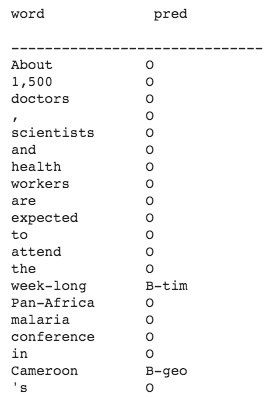The week-long is predicted as a timing tag (B-tim), which is correct. Cameroon is predicted as a geographical (B-geo) entity, and we know that Cameroon is a country in South Africa, and it is a Geographical entity. It has predicted Yaounde is an organization tag, but this prediction is not correct as Yaounde is the capital of Cameroon, so it should have been a geographical entity.

### Summary

In this short tutorial, you learned about developing a Deep Learning model for Named Entity Recognition. The model mainly used a bidirectional LSTM to learn the sequence of words in a sentence. You used the GMB text corpus: a huge repository of sentences with words marked with the BIO scheme. The model has several applications in NLP, with one of the applications being News Aggregation. Another approach to this problem could be using bidirectional LSTM with CRF (Conditional Random Fields). We will cover this in some other tutorial.